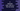# C program to separate even and odd numbers from an array### C program to separate even and odd numbers from an array and put in different array :

In this example, we will learn how to separate odd and even numbers from an array and put it in different array. The steps we are going to use in this program is as below :

### Algorithm :

1. One array with few numbers are given.
2. First create two more array to store odd and even numbers.
3. Run one loop and check for each number if it is divisible by 2 or not.
4. If yes, put it in the array for even numbers. Else, put it in the array for odd numbers.
5. Print out the odd and even numbers arrays.

### C program :

``````#include<stdio.h>

int main()
{
//1
int i,j,k;

//2
int NUM = {1,2,3,4,5,6,7,8,9,10};

//3
int ODD;
int EVEN;

//4
j = 0;
k = 0;

for(i = 0; i<10 ; i++){
//5
if(NUM[i]%2 == 0){
EVEN[j] = NUM[i];
j++;
}else{
ODD[k] = NUM[i];
k++;
}
}

//6
printf("Even numbers : ");
for(i = 0;i<j; i++){
printf("%d ",EVEN[i]);
}

printf("\nOdd numbers : ");
for(i = 0;i<k; i++){
printf("%d ",ODD[i]);
}

printf("\n");
}``````

### Explanation :

The commented numbers in the above program denotes the steps below :

1. Create variables i,j and k.
2. Array NUM holds all the numbers. We will separate out odd and even numbers from this array.
3. Create two more array to store odd and even numbers : ODD and EVEN.
4. Initialize j and k as 0. j indicates current index for the array ODD and k indicates current index for the array EVEN. Run one for loop to scan all the numbers of the main array NUM.
5. Check for each numbers in the array NUM. If the number is even, add it to the EVEN array. Otherwise, add it to ODD array. If added to the EVEN array, increment j. Else, increment k.
6. After all numbers are scanned, print both odd and even numbers . That means, using a for loop, scan both EVEN and ODD array and print out all the containing numbers.

### Output :

``````Even numbers : 2 4 6 8 10
Odd numbers : 1 3 5 7 9``````

You might also like :

### Where is the color and why codevscolor ?

Long story short, I love paintings and I paint on weekends. We(me and my wife) have one Youtube channel. Don't forget to subscribe ❤️❤️🤩